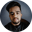Related Tags

sql
communitycreator
duplicate values

# How to find duplicate values in an SQL tableShubham Singh Kshatriya

In SQL, we can follow the following two steps to find duplicate values:

1. Group all the rows using the target column(s).
2. Check if the count of any group is greater than 1. Groups whose count is greater than 1 have duplicate values.

Let's look at an example.

### Example

First, let's create a table and insert some values into it.

/* Create table Student */
CREATE TABLE Student(
ID   INT              NOT NULL,
NAME VARCHAR (20)     NOT NULL,
AGE  INT              NOT NULL,
PRIMARY KEY (ID)
);

/* Insert values in table Student */
INSERT INTO Student (ID, NAME, AGE)
VALUES (1, 'Shubh', 22);

INSERT INTO Student (ID, NAME, AGE)
VALUES (2, 'Parth', 21);

INSERT INTO Student (ID, NAME, AGE)
VALUES (3, 'Shubh', 22);

INSERT INTO Student (ID, NAME, AGE)
VALUES (4, 'Pratik', 23);

/* Display table data */
SELECT * FROM Student;

### Explanation

• Lines 2–7: We create a table Student.
• Lines 10–20: We insert a few values in the Student table.
• Line 23: We display the data present in the Student table.

Note that we have added a duplicate value in the table.

Now let's find the duplicate values in this table using the column NAME as the target column. For clarity's sake, we repeat the steps above to recreate the table Student and then find the duplicate values in it.

/* Create table Student */
CREATE TABLE Student(
ID   INT              NOT NULL,
NAME VARCHAR (20)     NOT NULL,
AGE  INT              NOT NULL,
PRIMARY KEY (ID)
);

/* Insert values in table Student */
INSERT INTO Student (ID, NAME, AGE)
VALUES (1, 'Shubh', 22);

INSERT INTO Student (ID, NAME, AGE)
VALUES (2, 'Parth', 21);

INSERT INTO Student (ID, NAME, AGE)
VALUES (3, 'Shubh', 22);

INSERT INTO Student (ID, NAME, AGE)
VALUES (4, 'Pratik', 23);

/* Finding the duplicate values in 'Student' table */
SELECT NAME, COUNT(NAME) FROM Student
GROUP BY NAME
HAVING COUNT(NAME) > 1;
Finding the duplicate values in a SQL table

### Explanation

• Lines 2–7: We create a table Student.
• Lines 10–20: We insert a few values in the Student table.
• Lines 23–25: We find the duplicate values in the Student table and display its NAME and COUNT.

### Output

In the output, we can see that the value Shubh has COUNT 2. Thus, we know that it's a duplicate value.

RELATED TAGS

sql
communitycreator
duplicate values

CONTRIBUTORShubham Singh Kshatriya
RELATED COURSES

View all Courses

Keep Exploring

Learn in-demand tech skills in half the time